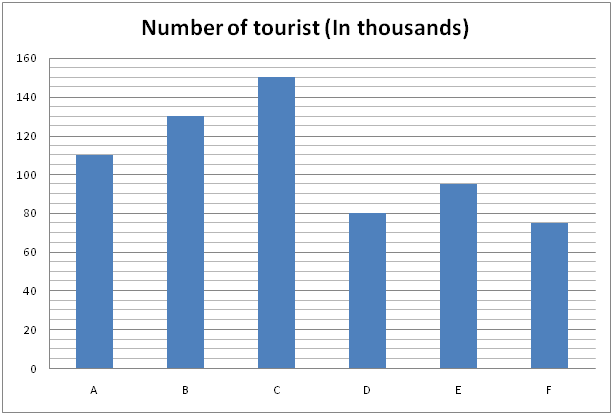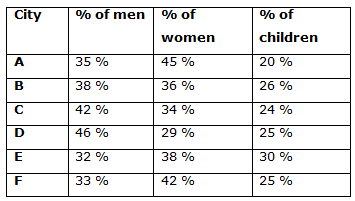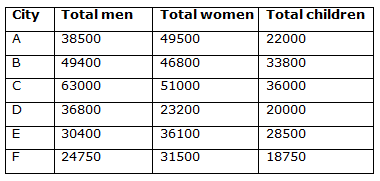# IBPS PO Mains 2018 – Quantitative Aptitude Questions Day-31

Dear Readers, Bank Exam Race for the Year 2018 is already started, To enrich your preparation here we have providing new series of Practice Questions on Quantitative Aptitude – Section. Candidates those who are preparing for IBPS PO Mains 2018 Exams can practice these questions daily and make your preparation effective.

[WpProQuiz 4322]

Directions (Q. 1 – 5): In each of these questions two equations (I) and (II) are given. You have to solve both the equations and give answer as,

a) If x > y

b) If x ≥ y

c) If x < y

d) If x ≤ y

e) If x = y or no relation can be established between x and y

1) I) 5x2 – 22x – 15 = 0

II) 4y2 – 24y – 13 = 0

2) I) 2x2 + 19x + 45 = 0

II) y2 – 17y + 72 = 0

3) I) x3 = 262 + 83 ÷ 16 × 4 + 196

II) y = (4096)1/4

4) I) 5x – 3y = 14

II) 2x – 5y = -21

5) I) 2x2 – 12x + 16 = 0

II) 3y2 + 4y – 15 = 0

Directions (Q. 6 – 10) Study the following information carefully and answer the given questions:

The following bar graph shows the number of tourist (in thousands) visited six different places in India in the year 2017 and the table shows the percentage of men, women and children’s among them.6) Find the total number of men tourist visited all the given cities together

a) 242850

b) 256730

c) 198640

d) 295460

e) None of these

7) Total number of women and children’s who are all visited the city A and D together is approximately what percentage more/less than the total number of men and children’s who are all visited the city B and E together?

a) 34 % less

b) 6 % more

c) 34 % more

d) 19 % less

e) 6 % less

8) Find the difference between the total number of men who are all visited the city B, C and F together to that of total number of women who are all visited the city A, D and E together?

a) 19870

b) 21740

c) 28350

d) 27560

e) None of these

9) Find the ratio between the total number of men to that of children’s who are all visited all the given cities together?

a) 4112: 2895

b) 4857: 3181

c) 3562: 2893

d) 5126: 3895

e) None of these

10) Total number of people who visited the city A, B and E together is approximately what percentage of the total number of people who visited the city C, D and F together?

a) 110 %

b) 125 %

c) 95 %

d) 80 %

e) 135 %

#### IBPS PO Prelims Quantitative Aptitude

Direction (1-5) :

I) 5x2 – 22x -15 = 0

5x2 – 25x + 3x -15 = 0

5x(x – 5) + 3(x – 5) = 0

(5x + 3) (x – 5) = 0

X = -3/5, 5 = -0.6, 5

II) 4y2 – 24y – 13 = 0

4y2 + 2y – 26y – 13 = 0

2y (2y + 1) – 13(2y + 1) = 0

(2y – 13) (2y + 1) = 0

Y = 13/2, -1/2 = 6.5, -0.5

Can’t be determined

I) 2x2 + 19x + 45 = 0

2x2 + 10x + 9x + 45 = 0

2x(x + 5) + 9 (x + 5) = 0

(2x + 9) (x + 5) = 0

X = -9/2, -5 = -4.5, -5

II) y2 – 17y + 72 = 0

y2 – 8y – 9y + 72 = 0

y (y – 8) – 9(y – 9) = 0

(y – 8) (y – 9) = 0

Y = 8, 9

X < y

I) x3 = 262 + 83 ÷ 16 × 4 + 196

x3 = 676 + (512/16)*4 + 196

x3 = 676 + 128 + 196 = 1000

x = 10

II) y = (4096)1/4

Y = 8

x > y

I) 5x – 3y = 14 –> (1)

II) 2x – 5y = -21 –> (2)

By solving the equation (1) and (2), we get,

x = 7, y = 7

x = y

I) 2x2 – 12x + 16 = 0

2x2 – 4x – 8x + 16 = 0

2x(x – 2) – 8(x – 2) = 0

(2x – 8) (x – 2) = 0

x = 4, 2

II) 3y2 + 4y – 15 = 0

3y2 + 9y – 5y – 15 = 0

3y (y + 3) -5(y + 3) = 0

(3y – 5) (y + 3) = 0

Y = 5/3, -3 = 1.66, -3

x > y

Direction (6-10) :The total number of men tourist visited all the given cities together

= > 38500 + 49400 + 63000 + 36800 + 30400 + 24750

= > 242850

Total number of women and children’s who are all visited the city A and D together

= > 49500 + 22000 + 23200 + 20000

= > 114700

Total number of men and children’s who are all visited the city B and E together

= > 49400 + 33800 + 30400 + 28500

= > 142100

Required % = [(142100 – 114700)/142100]*100

= > 19.28 % = 19 % less

The total number of men who are all visited the city B, C and F together

= > 49400 + 63000 + 24750 = 137150

The total number of women who are all visited the city A, D and E together

= > 49500 + 23200 + 36100 = 108800

Required difference = 137150 – 108800 = 28350

The total number of men who are all visited all the given cities together

= > 38500 + 49400 + 63000 + 36800 + 30400 + 24750

= > 242850

The total number of children’s who are all visited all the given cities together

= > 22000 + 33800 + 36000 + 20000 + 28500 + 18750

= > 159050

Required ratio = 242850: 159050 = 4857: 3181

Total number of people who visited the city A, B and E together

= > 110000 + 130000 + 95000

= > 335000

Total number of people who visited the city C, D and F together

= > 150000 + 80000 + 75000

= > 305000

Required % = (335000/305000)*100 = 110 %

Daily Practice Test Schedule | Good Luck

 Topic Daily Publishing Time Daily News Papers & Editorials 8.00 AM Current Affairs Quiz 9.00 AM Current Affairs Quiz (Hindi) 9.30 AM IBPS Clerk Prelims – Reasoning 10.00 AM IBPS Clerk Prelims – Reasoning (Hindi) 10.30 AM IBPS Clerk Prelims – Quantitative Aptitude 11.00 AM IBPS Clerk Prelims – Quantitative Aptitude (Hindi) 11.30 AM Vocabulary (Based on The Hindu) 12.00 PM IBPS Clerk Prelims – English Language 1.00 PM SSC Practice Questions (Reasoning/Quantitative aptitude) 2.00 PM IBPS PO/Clerk – GK Questions 3.00 PM SSC Practice Questions (English/General Knowledge) 4.00 PM Daily Current Affairs Updates 5.00 PM IBPS PO Mains – Reasoning 6.00 PM IBPS PO Mains – Quantitative Aptitude 7.00 PM IBPS PO Mains – English Language 8.00 PM